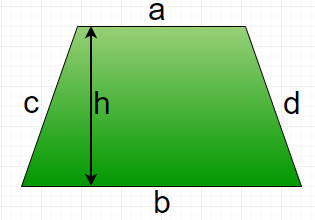# Program to calculate area and perimeter of Trapezium

• Last Updated : 22 Mar, 2021

A trapezium is a quadrilateral with at least one pair of parallel sides, other two sides may not be parallel. The parallel sides are called the bases of the trapezium and the other two sides are called it’s legs. The perpendicular distance between parallel sides is called height of trapezium.Formula :

```Area of Trapezium : 0.5 * (a + b) * h
Perimeter of Trapezium : a + b + c + d```

Examples :

```Input :  a = 5, b = 6, c = 4, d = 3, h = 8
Output : Area of Trapezium : 44
Perimeter of Trapezium : 18

Input :  a = 10, b = 15, c = 14, d = 11, h = 21
Output : Area of Trapezium: 262.5
Perimeter of Trapezium: 50```

Below is the implementation of above formula :

## C++

 `// CPP program to find area``// and perimeter of trapezium``#include ``using` `namespace` `std;` `// Function to calculate Area of trapezium``float` `areaTrapezium(``float` `a, ``float` `b, ``float` `h)``{``    ``return` `(1.0 / 2 * (a + b) * h);``}` `// Function to calculate perimeter of trapezium``float` `perimeterTrapezium(``float` `a, ``float` `b, ``float` `c,``                                           ``float` `d)``{``    ``return` `(a + b + c + d);``}` `// Driver function``int` `main()``{``    ``float` `a = 5, b = 15, c = 11, d = 4, h = 20;``    ``cout << ``"Area of Trapezium = "` `<<``                ``areaTrapezium(a, b, h) << endl;``    ``cout << ``"Perimeter of Trapezium = "` `<<``                ``perimeterTrapezium(a, b, c, d);``    ``return` `0;``}`

## Java

 `// Java program to calculate area``// and perimeter  of Trapezium` `public` `class` `GFG {``  ` `    ``// Function to calculate area of Trapezium``    ``public` `static` `float` `areaTrapezium (``float` `a,``                                ``float` `b, ``float` `h)``    ``{``        ``return` `((a + b) * h) / ``2``;``    ``}``    ` `    ``// Function to perimeter of Trapezium``    ``public` `static` `float` `perimeterTrapezium (``float` `a,``                            ``float` `b, ``float` `c, ``float` `d)``    ``{``        ``return` `(a + b + c + d);``    ``}` `    `  `// Driver function``public` `static` `void` `main(String args[])``{` `    ``// a, b, c, d are four sides of Trapezium``    ``// and h is height between two parallel sides.``    ``float` `a = ``5``;``    ``float` `b = ``15``;``    ``float` `c = ``11``;``    ``float` `d = ``4``;``    ``float` `h = ``20``;` `    ``// Printing value of  area.``    ``System.out.print(``"Area Of Trapezium : "``);``    ``System.out.println(areaTrapezium (a, b, h));``    ` `    ``// Printing value of  Perimeter.``    ``System.out.print(``"Perimeter Of Trapezium : "``);``    ``System.out.println(perimeterTrapezium (a, b, c, d));``}` `}` `// This code is contributed by "akanshgupta"`

## Python3

 `# Python3 code to find area``# and perimeter of trapezium` `# Function to calculate``# Area of trapezium``def` `areaTrapezium (a, b, h):``    ``return` `(``1.0` `/` `2` `*` `(a ``+` `b) ``*` `h)` `# Function to calculate``# perimeter of trapezium``def` `perimeterTrapezium (a, b, c, d):``    ``return` `(a ``+` `b ``+` `c ``+` `d)` `# Driver function``a ``=` `5``b ``=` `15``c ``=` `11``d ``=` `4``h ``=` `20``print``(``"Area of Trapezium ="``,``      ``areaTrapezium(a, b, h))` `print``(``"Perimeter of Trapezium ="``,``      ``perimeterTrapezium(a, b, c, d))` `# This code is contributed by "Sharad_Bhardwaj"`

## C#

 `// C# program to calculate area``// and perimeter of Trapezium``using` `System;` `class` `GFG {` `    ``// Function to calculate area of Trapezium``    ``public` `static` `float` `areaTrapezium (``float` `a,``                                 ``float` `b, ``float` `h)``    ``{``        ``return` `((a + b) * h) / 2;``    ``}``    ` `    ``// Function to perimeter of Trapezium``    ``public` `static` `float` `perimeterTrapezium (``float` `a,``                            ``float` `b, ``float` `c, ``float` `d)``    ``{``        ``return` `(a + b + c + d);``    ``}` `    `  `    ``// Driver function``    ``public` `static` `void` `Main()``    ``{``    ` `        ``// a, b, c, d are four sides of Trapezium``        ``// and h is height between two parallel sides.``        ``float` `a = 5;``        ``float` `b = 15;``        ``float` `c = 11;``        ``float` `d = 4;``        ``float` `h = 20;``    ` `        ``// Printing value of area.``        ``Console.Write(``"Area Of Trapezium : "``);``        ``Console.WriteLine(areaTrapezium (a, b, h));``        ` `        ``// Printing value of Perimeter.``        ``Console.Write(``"Perimeter Of Trapezium : "``);``        ``Console.WriteLine(perimeterTrapezium (a, b, c, d));``    ``}` `}` `// This code is contributed by "vt_m"`

## PHP

 ``

## Javascript

 ``

Output:

```Area of Trapezium = 200
Perimeter of Trapezium = 35```

My Personal Notes arrow_drop_up